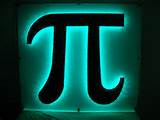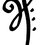# The History of PiIt's hard to pinpoint who, exactly, first became conscious of the constant ratio between the circumference of a circle and its diameter, though human civilizations seem to have been aware of it as early as 2550 BC. The Great Pyramid at Giza, which was built between 2550 and 2500 BC, has a perimeter of 1760 cubits and a height of 280 cubits, which gives it a ratio of 1760/280, or approximately 2 times pi. (One cubit is about 18 inches, though it was measured by a person's forearm length and thus varied from one person to another.) Egyptologists believe these proportions were chosen for symbolic reasons, but, of course, we can never be too sure. The earliest textual evidence of pi dates back to 1900 BC; both the Babylonians and the Egyptians had a rough idea of the value. The Babylonians estimated pi to be about 25/8 (3.125), while the Egyptians estimated it to be about 256/81 (roughly 3.16). . The Ancient Greek mathematician Archimedes of Syracuse (287-212 BC) is largely considered to be the first to calculate an accurate estimation of the value of pi. He accomplished this by finding the areas of two polygons: the polygon that was inscribed inside a circle, and the polygon in which a circle was circumscribed Archimedes didn't calculate the exact value of pi, but rather came up with a very close approximation—he used 96-sided polygons to come up with a value that fell between 3.1408 and 3.14285.

Chinese mathematician Zhu Chongzhi (AD 429-500) used a similar method to approximate the value of pi, using a 12,288-sided polygon. His best approximation was 355/113. The approximate ratio for pi also appears in the Bible in 1 Kings 7:23: "And he made a molten sea, ten cubits from the one brim to the other: it was round all about, and his height was five cubits: and a line of thirty cubits did compass it round about." (I should point out that the Biblical ratio for pi could be more accurate than one might think, since cubits changed depending on a person's forearm length. So, assuming the Bible isn't quoting cubits from the same person each time…) In the 15th century, Indian mathematician Madhavan of Sangamagramam discovered what is now known as the Madhava-Leibniz series (named after German mathematician Gottfried Leibniz, who rediscovered the series in the 17th century), an infinite series that converges to four. Madhavan subsequently calculated pi to 11 decimal places. Later, in 1707, Welsh mathematician William Jones was the first to use the Greek letter pi (π) to denote the constant ratio, though it wasn't until 1737 that Swiss mathematician and physicist Leonhard Euler popularized the use of the symbol. The π symbol was taken from the Greek word for "perimeter." Shigeru Kondo carried out the longest calculation of pi to date on October 19, 2011. The most accurate calculation of pi before the advent of the computer was by D. F. Ferguson, who calculated pi to 620 digits in 1945 (previously, William Shanks had calculated pi to 707 digits in 1874, but only 527 of said digits were correct). Of course, then computers entered the picture and calculating pi knew no bounds—beginning with D. F. Ferguson's calculation of pi to 710 digits in 1947 with a desk calculator to Takahashi Kanada's calculation of pi to 206,158,430,000 digits in 1999 with a Hitachi SR8000. Shigeru Kondo carried out the longest calculation of pi to date on October 19, 2011. Kondo used Alexander Yee’s y-cruncher program to calculate pi to 10 trillion digits, which is a record calculation for both supercomputers and home computers. So there you have it—Egyptians calculated pi using pyramids (or did they calculate pyramids using pi?), Archimedes busted out the 96-gon, Zhu Chongzhi one-upped him with a 12,288-gon (or is that 12,192-upped him?), Ferguson calculated 620 digits by hand, and Kondo used a supercomputer to nab the current world record of 10 trillion digit This is the history of pi please share your comments about this article.Note by Vishwathiga Jayasankar
6 years, 9 months ago

This discussion board is a place to discuss our Daily Challenges and the math and science related to those challenges. Explanations are more than just a solution — they should explain the steps and thinking strategies that you used to obtain the solution. Comments should further the discussion of math and science.

When posting on Brilliant:

• Use the emojis to react to an explanation, whether you're congratulating a job well done , or just really confused .
• Ask specific questions about the challenge or the steps in somebody's explanation. Well-posed questions can add a lot to the discussion, but posting "I don't understand!" doesn't help anyone.
• Try to contribute something new to the discussion, whether it is an extension, generalization or other idea related to the challenge.

MarkdownAppears as
*italics* or _italics_ italics
**bold** or __bold__ bold
- bulleted- list
• bulleted
• list
1. numbered2. list
1. numbered
2. list
Note: you must add a full line of space before and after lists for them to show up correctly
paragraph 1paragraph 2

paragraph 1

paragraph 2

[example link](https://brilliant.org)example link
> This is a quote
This is a quote
    # I indented these lines
# 4 spaces, and now they show
# up as a code block.

print "hello world"
# I indented these lines
# 4 spaces, and now they show
# up as a code block.

print "hello world"
MathAppears as
Remember to wrap math in $$ ... $$ or $ ... $ to ensure proper formatting.
2 \times 3 $2 \times 3$
2^{34} $2^{34}$
a_{i-1} $a_{i-1}$
\frac{2}{3} $\frac{2}{3}$
\sqrt{2} $\sqrt{2}$
\sum_{i=1}^3 $\sum_{i=1}^3$
\sin \theta $\sin \theta$
\boxed{123} $\boxed{123}$

Sort by:

Nice article!]

- 6 years, 5 months ago